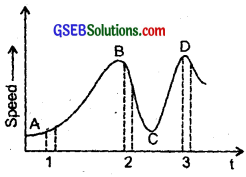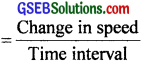# GSEB Solutions Class 11 Physics Chapter 3 Motion in a Straight Line

Gujarat Board GSEB Textbook Solutions Class 11 Physics Chapter 3 Motion in a Straight Line Textbook Questions and Answers.

## Gujarat Board Textbook Solutions Class 11 Physics Chapter 3 Motion in a Straight Line

### GSEB Class 11 Physics Motion in a Straight Line Text Book Questions and Answers

Question 1.
In which of the following examples of motion can the body be considered approximately a point object:
(a) a railway carriage moving without jerks between two stations.
(b) a monkey sitting on the top of a man cycling smoothly on a circular track.
(c) a spinning cricket ball that turns sharply on hitting the ground.
(d) a tumbling beaker that has slipped off the edge of a table?
In (a) and (b), we can consider the body approximately as a point object because the motion of the object involves changes of position by distance much greater than its size.Question 2.
The position, time (x – t) graphs for two children A and B returning from their school O to their homes P and Q respectively are shown in figure 3.1. Choose the correct entries in the brackets below:
(a) (A/B) lives; closer to the school than (B/A)
(b) (A/B) starts from school earlier than (B/A)
(c) (A/B) walks faster than (B/A)
(d) A and B reach home at the (same/different) time.
(e) (A/B) overtakes (B/A) on the road (once/twice).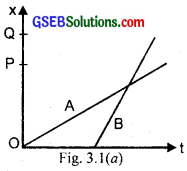(a) It is clear from the graph that OQ > OP, so A lives closer to the school than B.

(b) The position-time (x – t) graph of A starts from the origin, so x = 0, t = 0 for A while the x – t graph of B starts from C which shows that B starts later than A after a time interval OC. So A starts from school (O) earlier than B.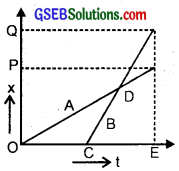(c) The speed is represented by the slope or steepness of the x – t graph. More steeper the graph, more will be the speed, so faster will be the child having steeper graph. As the x – t graph of B is steeper than the x – t graph of A, so we conclude that B walks faster than A.

(d) Corresponding to P and Q, the value of t from x – t graphs for A and B is same i.e. OE. So both A and B reach borne at the same time.

(e) As the x – t graphs for A and B intersect each other at one point i.e. D and B starts from the school later, so B over takes A on the road only once.Question 3.
A Woman starts from her home at 9.00 a.m., walks with a speed of 5 kmh-1 on a straight road up to her office 2.5 km away, stays at the office up to 5.00 p.m. and returns home by an auto with a speed of 25 kmh-1. Choose suitable scales and plot the x – t graph of her motion?
x – t graph of the motion of woman is shown in fig. 3.2 v1 = speed of woman while walking at 5kmh-1
x = Distance covered by her = 2.5 km
If t1 = time taken to reach office, then it can be calculated by using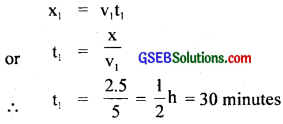If O is regraded as origin for both time and distance, then at t = 9.00 a.m., x = 0 at t = 9.30 a.m., x = 2.5 km and she reaches in her office.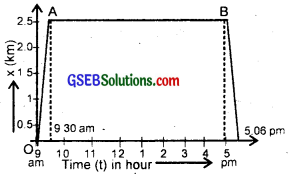When she stays at her office from 9.30 a.m. to 5.00 p.m., then she is stationary and hence her stay is represented by the straight line AB in the graph.
On return, speed of auto, v2 = 25 km/h.
∴ If t2 = time taken by her i.e. by auto from office to her home then
t2 = $$\frac{x}{v_{2}}$$ = $$\frac{2.5}{25}$$ = $$\frac{1}{10}$$h = 6 minutes.
Thus she reaches back to her home at 5.06 p.m.
Her motion on the return journey in shown by BC part of the graph.

Scale chosen:
Time on x-axis, 1 division = 1 hour.
Distance on y axis, 1 division = 0.5 km.

Question 4.
A drunkard walking in a narrow lane takes 5 steps forward and 3 steps backward, followed again by 5 steps forward and 3 steps backward and so on. Each step is 1 m long and requires Is. Plot the x – t graph of his motion. Determine graphically and otherwise how long the drunkard takes to fall in a pit 13 m away from the start.
The x – t graph of the drunkard is shown in Fig. 3.3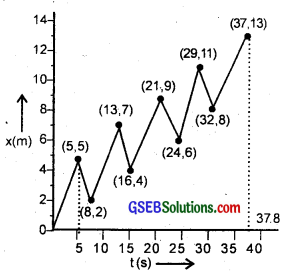Length of each step = 1m, time taken for each step = 1s.
∴ Time taken to move by 5 steps = 5s.
5 steps (i.e. 5m) forward and 3 steps (i.e. 3m) backward means that the net distance covered by him in first 8 steps i.e. in 8s = 5 m – 3 m = 2 m.
Distance covered by him in first 16 steps or 16s = 2 + 2 = 4 m.
Distance covered by the drunkard in first 24s i.e. 24 steps = 2 + 2 + 2 = 6 m.
and Distance covered in 32 steps i.e. 32 sec. = 8 m.
∴ Distance covered in first 37 steps = 8 + 5 = 13 m.
Distance of the pit from the start = 13 m.
∴ Total time taken by the drunkard to fall in the pit = 37 s.
Since 1 step requires Is of time, so we arrive at the same result from the graph shown.Question 5.
A jet airplane travelling at a speed of 500 kmh-1 ejects its products of combustion at the speed of 1500 kmh-1 relative to the jet plane. What is the speed of the latter with respect to an observer on the ground?
Let Vi, Vg and V0 be the velocities of jet, ejected gases i.e. combustion products and observer on the ground respectively.
Let jet be moving towards right (+ve direction).
∴ ejected gases will move towards left (-ve direction).
∴ According to the statement
vi = 500 kmh-1
As observer is at ground i.e. at rest ”
∴ v0 = 0
Now relative velocity of plane w.r.t. the observer
vg – vi = 500 – 0
= 500 kmh-1 …………………. (i)
Relative velocity of the combustion products w.r.t. jet plane
vg – vi = -1500 kmh-1 (given) ……………… (ii)
– ve sign indicates that the combustion products move in a direction opposite to that of jet.
∴ Adding equations (i) and (ii), we get the speed of combustion products w.r.t. observer on the ground i.e.
(vj – v0) + (vg – vj) = vg – v0 = 500 + (-1500)
vg – v0 = -1000 kmh-1
– ve sign shows that relative velocity of the ejected gases w.r.t. observer is towards left i.e. – ve direction i.e. in a direction opposite to the motion of the jet plane.

Question 6.
A car moving along a straight highway with speed of 126 kmh-1 is brought to a stop within a distance of 200 m. What is the retardation of the car (assumed uniform) and how long does it take for the car to stop?
Initial velocity of car,
= 126 kmh-1
= 126 × $$\frac{5}{18}$$ms-1 = 35ms-1 ………………… (i)
As the car finally comes to rest, v = 0
Distance covered, s = 200 m, a = ?, t = ?
We know that v2 – u2 = 2as,
∴ a = $$\frac{v^{2}-u^{2}}{2 s}$$ ……………… (ii)
putting the values from equation (i) in equation (ii), we get
a = $$\frac{0-(35)^{2}}{2 \times 200}$$ = $$\frac{35×35}{200}$$
– ve sign shows that acceleration in negative which is called retardation i.e. car is uniformally retarded at, – a = 3.06 ms2
To find t, let us use the relation,
v = u + at
Here, a = – 3.06 ms-2, v = 0, u = 35 ms-1
∴ t = $$\frac{v-u}{a}$$ = $$\frac{0-35}{-3.06}$$ = 11.44 s
∴ t = 11.44 sec.Question 7.
Two trains A and B of length 400 m each are moving on two parallel tracks with a uniform speed of 72 kmh-1 in the same direction, with A ahead of B. The driver of B decides to overtake A and accelerates by 1 ms-2. If after 50s, the guard of B just brushes past the driver of A, what was the original distance between them?
Originally both the trains A and B have the same velocities,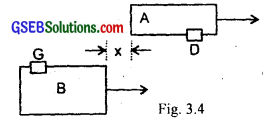i.e. if uA and uB be the initial speeds of the two trains, then
uA = uB = 72 kmh-1 = 72 × $$\frac{5}{18}$$ = 20ms-1
Let x = original distance between the two trains
Now for train B, a = 1 ms-2, t = 50 s.
Let SB be the distance covered by train B in 50 s, Then.
Using the relation S = ut + $$\frac{1}{2}$$ at2, we get
SB = uBt + $$\frac{1}{2}$$ at2
= 20 × 50 + $$\frac{1}{2}$$ × 1 × (50)2
= 1000 + 1250 = 2250 m.
Also let SA be the distance covered by the train A during 50 s then from the relation,
x = ut,
we get SA = uA × t
or SA = 20 × 50 = 1000 m.
Original distance between the two trains = SB – SA
= 2250 – 1000
= 1250 m.

Aliter:
As originially both the trains are moving with the same velocities, so relative velocity of B w.r.t. A is = 0
ie. VBA = 0.
Similarly VAB = 0
Now for train B, a = 1 ms-2, VBA = 0, t = 50 s.
x(t) – x(0) = ?
We know that
x(t) – x(0) = VBAt + $$\frac{1}{2}$$at2
= 0 × 50 + $$\frac{1}{2}$$ × 1 × (50)2
= 1250 m.Question 8.
On a two lane road, car A is travelling with a speed of 36 kmh-1. Two cars B and C approach car A in opposite directions with a speed of 54 kmh-1 each. At a certain instant, when the distance AB is equal to AC, both being 1 km, B decides to overtake A before C does. What minimum acceleration of car B is required to avoid an accident?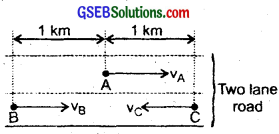Speed of car A, VA = VA = 36 × $$\frac{5}{18}$$ = 10 ms-1
Let VB = VC = 54 kmh-1
= 54 × $$\frac{5}{18}$$ = 15ms-1 (given)

∴ Relative speed of car B w.r.t. car A i.e; VBA is given by
VBA = VB – VA
= 15 – 10 = 5 ms-1

Also Relative speed of car C w.r.t. car A i.e; VCA is given by
VCA = VC – (-VA)
= VC + VA
= 15 + 10 = 25 ms-1

Also AB = AC = 1 km (given) = 1000 m
Let t = time taken by car AC to travel distance AC
∴ using relation S = ut (∴ car C is in uniform motion)
we get t = $$\frac{S}{u}$$ = $$\frac{\mathrm{AC}}{\mathrm{V}_{\mathrm{CA}}}$$ = $$\frac{1000}{25}$$ = 40s.
Let a = acceleration of car B for t = 40s; so it will cover 1000 m in 40s.
∴ using the relation, S = ut + $$\frac{1}{2}$$at2, we get
AB = VBAt + $$\frac{1}{2}$$at2
or 1000 = 5 × 40 + $$\frac{1}{2}$$ a × (40)2
= 200 + a × $$\frac{1600}{2}$$
or 800 = 800
a = 1 ms2.

Question 9.
Two towns A and B are connected by a regular bus service with a bus leaving in either direction every T minutes. A man cycling with a speed of 20 kmh-1 in the direction A to B notices that a bus goes past him every 18 minutes in the direction of his motion and every 6 minutes in opposite direction. What is the period T of the bus service and with what speed (assumed constant) do the buses ply on the road?
Let the speed of each bus = vb. kmh-1
and Speed of cyclist = vc = 20 kmh-1

Case I:
Relative speed of the buses plying in the direction of motion of cyclist i.e. from A to B = vb – vc = (vb – 20) kmh-1
Since the bus goes past the cyclist every 18 minutes (= $$\frac{18}{60}$$h),
∴ Distance covered by the bus w.r.t. the cyclist
= (vb – 20) × $$\frac{18}{60}$$ km …………………… (i)
Since the bus leaves after every T minutes, so the distance covered by the bus in T min (= $$\frac{T}{60}$$ h) is given by
= vb × $$\frac{T}{60}$$ ………………….. (ii)
∴ from (i) and (ii), we get
(vb – 20) × $$\frac{18}{60}$$ = vb × $$\frac{T}{60}$$
or vb – 20 = vb × $$\frac{T}{18}$$ ……………………. (iii)

Case II:
Relative speed of the bus coming from town B to A w.r.t. cyclist (vb + 20) kmh-1
(∵ cyclist is moving from A to B)
∴ Since the bus goes past the cyclist after every 6 minutes.
Distance covered by the bus w.r.t. the cyclist
= (vb + 20) × $$\frac{6}{60}$$ km ……………….. (iv)
Also distance covered by bus in T minutes is
= vb × $$\frac{T}{60}$$ km …………………… (v)
∴ Equating (iv) and (v), we get
(vb + 20) × $$\frac{6}{60}$$ = vb × $$\frac{T}{60}$$
or vb + 20 = vb × $$\frac{T}{6}$$ ………………… (vi)
Dividing (vi) by (iii), we get
$$\frac{v_{b}+20}{v_{b}-20}$$ = 3
or vb + 20 = 3 vb – 60
or 2Vb = 80
or vb = 40 kmh-1
putting the value of vb in equation (iii), we get
40 – 20 = 40 × $$\frac{T}{18}$$
or 20 = 40 × $$\frac{T}{18}$$
or T = 20 × $$\frac{18}{40}$$ = 9 minutes
∴ vb = 40kmh-1, T = 9 minutes.Question 10.
A player throws a ball upwards with an initial speed of 29.4 ms-1?
(a) What is the direction of acceleration during the upward motion of the ball?
(b) What are the velocity and acceleration of the ball at the highest point of its motion?
(c) Choose the x = 0 m and t0 = 0 s to be the location and time of the ball at its highest point, vertically downward direction to the positive direction of x-axis, and give the signs of position, velocity and acceleration of the ball during its, upward and downward motion.
(d) To what height does the ball rise and after how long does the ball return to the player’s hand? (Take g = 9.8 ms-2 and neglect air resistance)
(a) Since the ball is moving under the effect of gravity, the direction of acceleration due to gravity is always vertically downwards.

(b) When the ball is at the highest point of its motion, its velocity becomes zero and the acceleration is equal to the acceleration due to gravity = 9.8 ms-2 in vertically downward direction.

(c) When the highest point is chosen as the location for x = 0 and t = 0 and vertically downward direction to be the positive direction of x-axis.
During upward motion, sign of position is negative, sign, of velocity negative and the sign of acceleration positive i.e. v < 0, a > 0.
During downward motion, sign of position is positive, sign of velocity is positive and the sign of acceleration is r also positive i.e. v > 0, a > 0.

(d) Let t = time taken by the ball to reach the highest point.
H = height of the highest point from the ground.
Taking vertically upward motion of the ball.
∴ initial velocity, u = -29.4 ms-1, a = g = 9.8 ms-2, Final velocity v = 0, s = H = ?, t = ?
Using the relation, v2 – u2 = 2as, we get
02 – (29.4)2 = 2 × 9.8 H
or H = $$\frac{-29.4×29.4}{2×9.8}$$ = -44.1 m
where -ve sign shows that the distance is covered in upward direction.
Using equations v = u + at, we get
0 = -29.4 + 9.8 × t
∴ t = $$\frac{29.4}{9.8}$$ = 3s
i.e. time of ascent = 3s.
Also we know that when the object moves under the effect of gravity alone, the time of ascent is always equal to the time of descent.
∴ Total time after which the ball returns to the player’s hand = 2t = 2 × 3 = 6s.Question 11.
Read each statement below carefully and state with reasons and examples, if it is true or false. A particle in one-dimensional motion?
(a) with zero speed at an instant may have non-zero acceleration at that instant.
(b) with zero speed may have non-zero velocity.
(c) with constant speed must have zero acceleration.
(d) with positive value of acceleration must be speeding up.
(a) True, example:- if the ball is in vertically upward motion i.e. thrown vertically upward, then it will have zero speed at the highest point and an acceleration of 9.8 ms-2 in downward direction.

(b) False, because speed is the magnitude of velocity.

(c) True, if the particle rebounds instantly with the same speed, so the acceleration will be zero.
Example: Elastic collision of a ball with an elastic floor.

(d) False, e.g. if we take the positive direction of acceleration due to gravity downwards, a ball thrown vertically up will have its speed decreasing. However, the statement can be true if the positive direction of acceleration is along the direction of motion.

Question 12.
A ball is dropped from a height of 90 m on a floor. At each collision with the floor, the ball loses one tenth of its speed. Plot the speed-time graph of its motion between t = 0 to 12s.
Here, initial velocity, u = 0
Height from which the ball is dropped, h = 90 m
Loss of its speed after every collision = $$\frac{1}{10}$$ i.e. 10% of its speed of hitting the ground
∴ Velocity of rise after each collision = velocity of hitting – its 10%
acceleration = a = g = 9.8 ms-2
If v1 and t1 be the velocity of rise and time taken in first hitting the floor, then using equations,
v2 – u2 = 2as ………………. (i)
and s = ut + $$\frac{1}{2}$$ at2 …………………. (ii)
We get
or $$v_{1}^{2}-0=2 g h$$
v1 = $$\sqrt{2gh}$$
= $$\sqrt{2×9.8×90}$$
= $$\sqrt{2×49×2×9}$$
= 42 ms-1
and h = $$\frac{1}{2}$$ gt12
or t1 = $$\frac{\sqrt{2h}}{g}$$
or = $$\frac{\sqrt{2×90}}{9.8}$$ = $$\frac{30}{7}$$ = 4.28s
∴ If v2 = v1 – 10% of v1 = 42 – 4.2 = 37.8 ms-1
∴ If v2 be the velocity of its first rise i.e. first rebounce,
v2 = v1 – 10% of v1 = 42 – 4.2 = 37.8 ms-1
If h1, be the height reached after first rebounce
then at h = h1, v3 = 0, v2 = u
∴ From equations (i), we get
$$0-v_{2}^{2}$$ = 2(-g) × h1
or $$\frac{v_{2}^{2}}{2 g}$$
= $$\frac{(37.8)^{2}}{2 \times 9.8}$$
= $$\frac{37.8×37.8}{2×9.8}$$
= 72.9 m.
The ball rises with v2 and returns back to ground with v3 (say), so v net displacement is zero i.e. S = 0
Then from (i), we get
or $$\mathbf{v}_{3}^{2}-\mathbf{v}_{2}^{2}$$ = 2g × 0
v3 = v2 = 37.8 ms-1
If t2 be the time elapsed between two successive hits,
Then using equation (if), we get
0 = v2t2 + $$\frac{1}{2}$$ (-g)t22
or 0 = 37.8 t2 – $$\frac{9.8}{2}$$ t22
or 4.9 t2 = 37.8
or t2 = $$\frac{37.8}{4.9}$$ = $$\frac{54}{7}$$ = 7.714 s.
Now let v4 = velocity of rise after second hit
= v3 – 10% of v3
= 37.8 – 3.78
= 34.02 ms-1
Now let v4 = velocity of rise after second hit
= v3 – 10% of v3
= 37.8 – 3.78
= 34.02 ms-1
Also, t2 = 7.714 s is the total time of flight
Also we know that time of ascent = time of descent = t (say)
∴ t2 = 2t
or t = $$\frac{7.714}{2}$$ = 3.857 = 3.86 s
Now t1 + t2 = 4.28 + 7.714 = 11.994 = 12 s.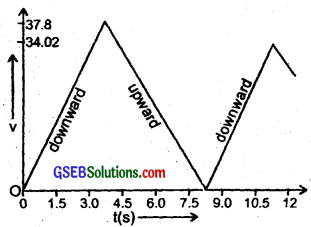Thus the ball rises only once after fall from 90 m with 37.8 ms-1 in the time interval from t = 0 to 12 s. So the plot of speed-time graph is as shown in fig. 3.6.
Also the velocity with which the ball hits the ground = 37.8 ms-1Question 13.
Explain clearly, with examples, the distinction between:
(a) magnitude of displacement (sometimes called distance) over an interval of time and the total length of path covered by a particle over the same interval.
(b) magnitude of average velocity over an interval of time and the average speed over the same interval. [Average speed of a particle over an interval of time is defined as the total path length divided by the time interval].
Show in both (a) and (A) that the second quantity is either greater than or equal to the first. When is the equality sign true? [For simplicity, consider one-dimensional motion only].
(a) Magnitude of displacement or distance travelled by a body in a given time interval is the difference between the initial and final positions of the body. It can be represented by |$$\vec{r}-\overrightarrow{r_{0}}$$|.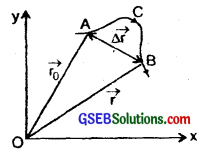The total length of the path covered by a body is the length of the actual path travelled by it. The total length of the path is either equal or greater than the magnitude of the displacement.

(b) Let a particle at times t1 and t2 be at the positions A and B respectively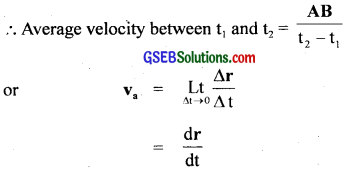where ∆t = t2 – t1
The average velocity does not tell us whether the motion of the particle was actually along the path AB or along ACB and whether the motion between t1 and t2 was steady or not. Average speed is defined as the ratio of the total distance covered by the body to the total time taken, e.g. Let a person goes to a temple 1 km away from his house and comes back taking 1 hour to perform the journey both ways.
∴ Average speed of the man = $$\frac{1+1}{1}$$ = 2 km/h.
But average velocity is zero because his displacement during the time of 1 hour is zero.
Thus in either case (a) and (b) the second quantity is either greater than or equal to the first quantity. Equality sign is true when displacement is equal to the distance.Question 14.
A man walks on a straight road from his home to a market 2.5 km away with a speed of 5 kmh-1. Finding the market closed, he instantly turns and walks back home with a speed of 7.5 kmh-1. What is the:
(a) magnitude of average velocity and
(b) average speed of the man over the interval of time
(i) 0 to 30 min
(ii) 0 to 50 min
(iii) 0 to 40 min?
[Note: You will appreciate from the exercise why it is better to define average speed as total path length divided by time, and not as magnitude of average velocity. You would not like to tell the tired man on his return home that his average speed was zero.]
We know that average velocity = image 12
and average speed = image 13

(i) 0 to 30 min interval:
Distance covered in going to market = 2.5 km
Speed = 5kmh-1
∴ Time taken to go to market = Distance/Speed
= 2.5/5
= $$\frac{1}{2}$$h = 30 min.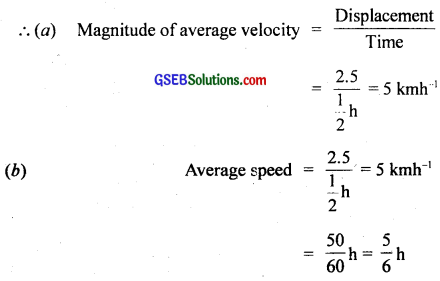(ii) 0 to 50 min interval
Speed of return journey = 7.5 kmh-1
Distance to be covered to reach home = 2.5 km
∴ time taken in return journey = image 14
= $$\frac{2.5}{7.5}$$ = $$\frac{1}{3}$$h
= 20 min.
∴ Total time taken for the journey = 30 + 20 = 50 min.
Now net displacement of the man is zero as he started from his home and come back home, i.e; 25 – 25 = 0 km.
∴ Magnitude of average velocity = $$\frac{0}{\frac{5}{6}}$$ = 0
Total distance covered during to whole journey = 2.5 + 2.5
= 5 km
∴ Average speed = $$\frac{5}{\frac{5}{6}}$$ = 6 kmh-1
= $$\frac{2}{3}$$h

(iii) 0 to 40 min interval
As the man takes 30 min to go to market
∴ time for which he performed return journey = 40 – 30
= 10 min.
Distance covered in 10 min = Velocity of return journey × time
= 7.5 × $$\frac{10}{60}$$
= 1.25 km
∴ Net displacement of the man = 2.5 – 1.25
= 1.25 km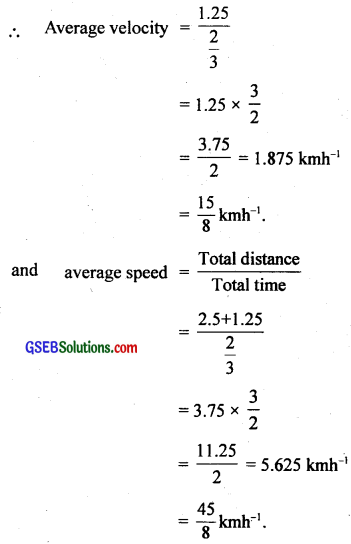Thus from case (ii), we see that average velocity is zero but not the average speed, so it is better to define average speed as the ratio of the total distance covered to the total time taken and not as the magnitude of average velocity.Question 15.
In Exercises 3.13 and 3.14 we have carefully distinguished between average speed and magnitude of average velocity. No such distinction is necessary when we consider instantaneous speed and magnitude of velocity. The instantaneous speed is alw ays equal to the magnitude of instantaneous velocity. Why?
The instantaneous velocity is defined as the velocity of an object at a particular instant of time.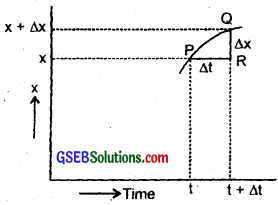The instantaneous speed is defined as the limiting value of the average speed. Thus when time interval is very small, the magnitude of the displacement is effectively equal to the distance travelled by the object in the same small interval of time. Thus both instantaneous velocity and instantaneous speed are equal in this case. This can be understood from the following as:
Consider a small displacement over a time At between time interval, t and t + ∆t.
∴ Average velocity in the interval ∆t = $$\frac{∆x}{∆t}$$
∴ Instantaneous velocity at instant t is = image 16
where arc PQ ~ length of the line PQ.
Now from ∆ PQR, PQ2 = PR2 + QR2
As ∆t → 0, PQ → QR or PQ → ∆x
∴ From (ii), we get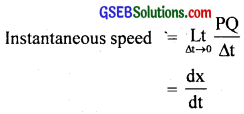magnitude of instantaneous velocity.

Question 16.
Look at the graphs (a) to (d) carefully and state with reasons, which of these cannot possible represent one-dimensional motion of a particle?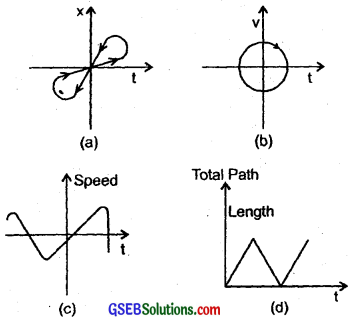(a) A line drawn for a given time parallel to position axis will cut the graph at two points which means that at a given instant of time, the particle will have two positions which is not possible. Hence graph (a) is not possible i.e. does not represent one dimensional motion.

(b) This graph does not represent one dimensional motion because at a give instant of time, the particle will have two values of velocity in positive, as well as in negative direction which is not possible in one dimensional motion.

(c) This is a graph between speed and time and does not represent one dimensional motion as this graph tells that the particle can have the negative speed but the speed can never be negative.

(d) This does not represent one dimensional motion, as this graph tells that the total path length decreases after certain time but total path length of a moving particle can never decrease with time.Question 17.
Figure below shows the x – t plot of one dimensional motion of a particle. Is it correct to say from the graph that the particle moves in a straight line for t < 0 and on a parabolic path for t > 0? If not, suggest a suitable physical context for this graph?
No, it is not correct to say that the particle moves in a straight line for t < 0 and on a parabolic path for t > 0 as the x – t graph does not show the trajectory of the path of a particle.
The graph shows that at time
t = 0, x = 0
Context: The suitable context is a body dropped from a tower i.e.
free fall of a body i.e. x = 0 at t = 0.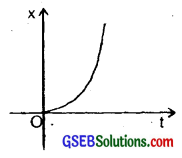Question 18.
A police van moving on a highway with a speed of 30 kmh-1 fires a bullet at a thief’s car speeding away in the same direction with a speed of 192 kmh-1. If the muzzle speed of the bullet is 150 ms-1, with what speed does the bullet hit the thief’s car? (Note : Obtain that speed which is relevant for damaging the thief’s car.)
Speed of thief’s car, vt = 192 kmh-1
= 192 × $$\frac{15}{18}$$ ms-1 = $$\frac{160}{3}$$ ms-1
Speed of police van, vp = 30 kmh-1
= 30 × $$\frac{5}{18}$$ = $$\frac{25}{3}$$ ms-1
vb = speed of the bullet with which it is actually fired = 150 ms-1
∴ speed of the bullet, vb = speed of police van + vb
= vp + vb
= $$\frac{25}{3}$$ + 150 = $$\frac{473}{3}$$ms-1
∴ Speed of bullet with which it hits the thief’s car is = Relative speed of bullet w.r.t. police van – relative speed of thief’s car = Vbt
i.e. Vbt – Vb – Vt = ($$\frac{473}{3}$$ – $$\frac{160}{3}$$)ms-1
= $$\frac{315}{3}$$ = 105 ms-1
Aliter: As above
Vt = 192 kmh-1 = $$\frac{160}{3}$$ ms-1
VP = 30 kmh-1 = $$\frac{25}{3}$$ ms-1
∴ Relative speed of thief’s car w.r.t. police van,
Vtp = Vt – Vp
= $$\frac{160}{3}$$ – $$\frac{25}{3}$$
= $$\frac{135}{3}$$ = 45ms-1
muzzle speed of bullet, Vb = 150 ms-1 = relative speed of bullet w.r.t. police van
∴ Speed of bullet with which ¡t hits the thief’s car = relative speed of bullet wr.r. police van – relative speed of thief’s car w.r.t. police van
= 150 – 45 = 105ms-1.Question 19.
Suggest a suitable physical situation for each of the following graphs?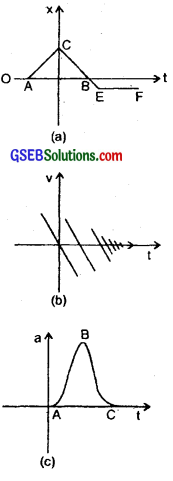(a) A ball at rest on a smooth floor is kicked. OA represents its rest position. At C the ball rebounds from the wall. CB represents the bounced ball with reduced speed because the slope of CB is less than that of AC. (The slope of x – t graph gives the speed of the moving body); EF shows that the ball is stopped by the other wall.

(b) The graph represents the case of a ball thrown up with some initial velocity and rebounding from the floor with reduced speed after each hit every time on the floor i.e. after every bounce.

(c) It is the acceleration-time graph. The graph represents a uniformly moving cricket ball turned back after hitting the bat for a very short time interval (represented by the portion ABC of the graph).

Question 20.
Figure gives the x – t plot of a particle executing one dimensional simple harmonic motion (You will learn about this motion in more details in Chapter 14). Give the signs of position, velocity and acceleration variables of the particle at t = 0.3s, 1.2s, -1.2s.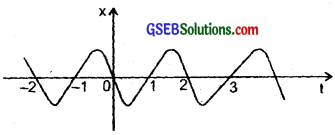We know that in S.H.M., the acceleration ‘a’ is given by the relation,
a = – ω2x …………………. (1)
where ω is the angular frequency and is a constant. Also velocity is given by, v = $$\frac{dx}{dt}$$ …………………. (2)
(i) At time t = 0.3s, x is negative, slope of x – t plot is negative, so position and velocity are negative but acceleration is positive according to equation (1).

(ii) At time, t = 1.2s, x is positive, the slope of x – t plot is also positive, hence position and velocity are positive but according to equation (1), acceleation is negative.

(iii) At time, t = – 1,2s, x is negative, the slope of x – t plot is also negative. But since both x and t are negative hence according to equation, (ii) velocity is positive. As position is negative, so according to equation (i) acceleration is positive.Question 21.
The following figure gives the x – t plot of a particle in one dimensional motion. Three different equal intervals of time are shown. In which interval is the average speed greatest, and in which is the least? Give the sign of average velocity for each interval?
We know that the average speed in a small time interval is equal to the slope of x – t graph in that interval of time. So the average speed is the greatest in the interval 3 because the slope is greatest and the average speed is least in the interval 2 because the slope is the least there. The average velocity is positive in the interval 1 and 2 and negative in the interval 3 as x2 – x1 is positive in 1, 2 intervals and negative in interval 3.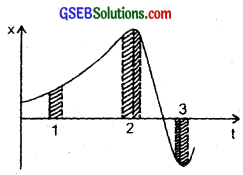Question 22.
Following figure gives a speed-time graph of a particle in motion along a constant direction. Three equal intervals of time are shown. In which interval is the average acceleration greatest in magnitude? In which interval is the average speed greatest? Choosing the positive direction as the constant direction of motion give the signs of v and a in the three intervals. What are the accelerations at the points A, B, C and D?